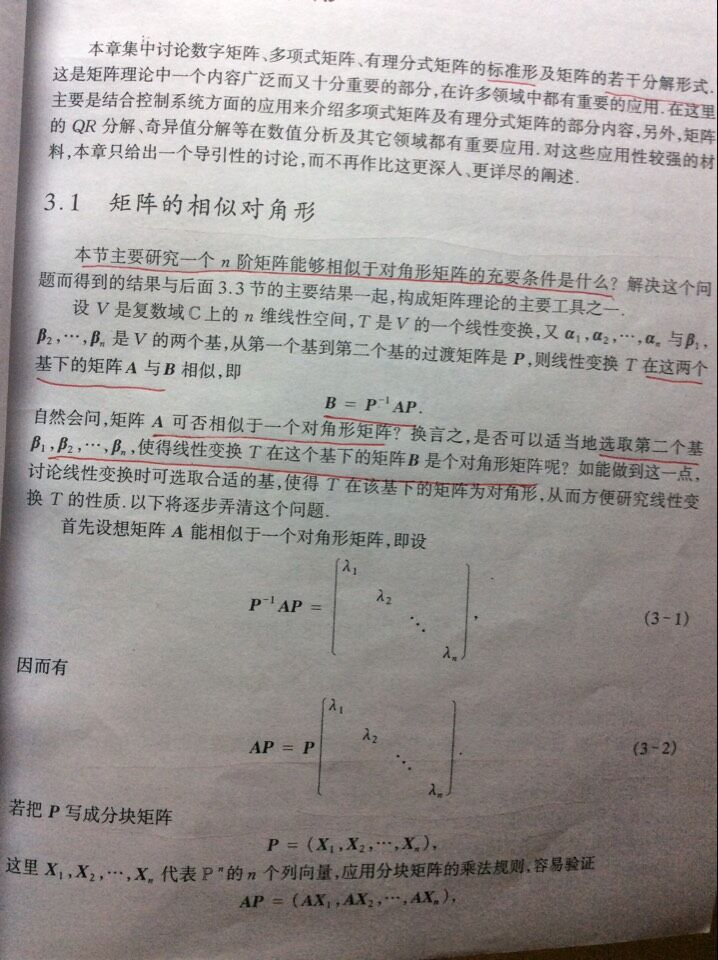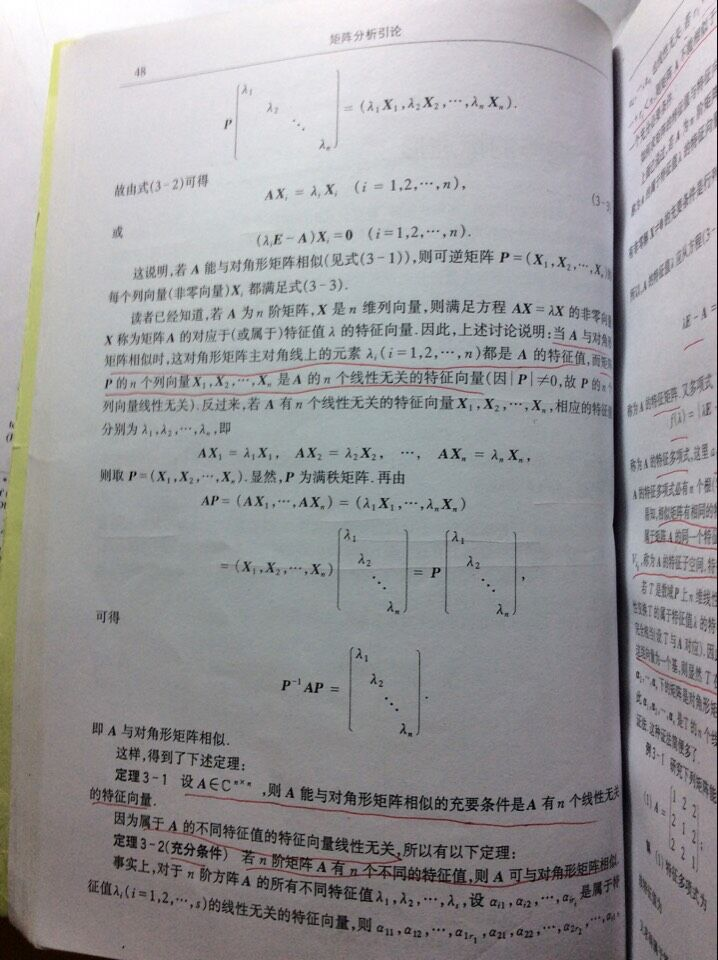• ## 循环矩阵傅里叶对角化

万次阅读 多人点赞 2016-03-15 14:10:30
“任意循环矩阵可以被傅里叶变换矩阵对角化”这个概念常常出现在论文中，本文其做简单解释。

All circulant matrices are made diagonal by the Discrete Fourier Transform (DFT), regardless of the generating vector x.
任意循环矩阵可以被傅里叶变换矩阵对角化。

文献中，一般用如下方式表达这一概念：
$X=C(x)=F \cdot diag(\hat{x}) \cdot F^H$
其中$X$是循环矩阵，$\hat{x}$是原向量$x$的傅里叶变换，$F$是傅里叶变换矩阵，上标H表示共轭转置：$X^H=(X^{*})^T$。
换句话说，$X$相似于对角阵，$X$的特征值是$\hat x$的元素。
另一方面，如果一个矩阵能够表示成两个傅里叶矩阵夹一个对角阵的乘积形式，则它是一个循环矩阵。其生成向量是对角元素的傅里叶逆变换：
$F \cdot diag(y) \cdot F^H = C(\mathcal{F}^{-1}(y))$
这个公式初看疑问很多，以下一一讨论。
$X$是什么？
$X$是由原向量$x$生成的循环矩阵。以矩阵尺寸$K=4$为例。
$X=C(x)=\begin{bmatrix} x_1 & x_2 & x_3 & x_4 \\ x_4 & x_1 & x_2 & x_3 \\ x_3 & x_4 & x_1 & x_2 \\ x_2 & x_3 & x_4 & x_1 \\ \end{bmatrix}$
$F$ 是什么？
$F$是离散傅里叶矩阵（DFT matrix），可以用一个复数$\omega = e^{-2\pi i/K}$表示，其中$K$为方阵$F$的尺寸。以$K=4$为例。
$F=\frac{1}{\sqrt{K}} \begin{bmatrix} 1 & 1 & 1 & 1 \\ 1 & \omega & \omega^2 & \omega^3 \\ 1 & \omega^2 & \omega ^4 & \omega^6 \\ 1 & \omega^3 & \omega^6 & \omega^9 \end{bmatrix}$
把$\omega$想象成一个角度为$2\pi/K$的向量，这个矩阵的每一行是这个向量在不断旋转。从上到下，旋转速度越来越快。
之所以称为DFT matrix，是因为一个信号的DFT变换可以用此矩阵的乘积获得：
$\hat{x}=DFT(x)=\sqrt{K}\cdot F \cdot x$
反傅里叶变换也可以通过类似手段得到：
$x=\frac{1}{\sqrt{K}}F^{-1}\hat{x}$
傅里叶矩阵有许多性质：

是对称矩阵，观察$\omega$的规律即可知；
满足$F^HF=FF^H=I$，也就是说它是个酉矩阵（unitary）。可以通过将$F^{H}$展开成$\omega^{H}$验证。

注意：$F$是常数，可以提前计算好，和要处理的$x$无关。
对角化怎么理解？
把原公式两边乘以逆矩阵：
$F^{-1} \cdot X \cdot (F^H)^{-1}=diag(\hat{x})$
利用前述酉矩阵性质：
$左边=F^{H}XF=diag(\hat{x})$
也就是说，矩阵$X$通过相似变换$F$变成对角阵$diag(\hat{x})$，即对循环矩阵$X$进行对角化。
另外，$F^HXF$是矩阵$X$的2D DFT变换。即傅里叶变换可以把循环矩阵对角化。
怎么证明？
可以用构造特征值和特征向量的方法证明（参看这篇论文1的3.1节），此处简单描述。
考察待证明等式的第k列：
$X\cdot f_k=\hat x_k\cdot f_k$
其中$f_k$表示DFT矩阵的第k列，$\hat x_k$表示傅里叶变换的第k个元素。等价于求证：$f_k$和$\hat x_k$是$X$的一对特征向量和特征值。
左边向量的第i个元素为：$left_i = \left[ x^i, f_k \right]$。$x_i$表示把生成向量$x$向右移动i位，$[]$表示内积。
内积只和两个向量的相对位移有关，所以可以把$f_k$向左移动i位：$left_i=\left[ x, f_k^{-i}\right]$。
DFT矩阵列的移位可以通过数乘$\omega$的幂实现：$f_k^i=f_0\cdot \omega^{ik}$。

举例：$K=3$，
$F=\frac{1}{\sqrt{K}} \begin{bmatrix} 1 & 1 & 1\\ 1 & \omega & \omega^2 \\ 1 & \omega^2 & \omega ^4 \end{bmatrix}$

利用$\omega^N=1$.
$f_1\cdot \omega =[1,\omega,\omega^2]\cdot \omega = [\omega, \omega^2,\omega^3] = [\omega, \omega^2, 1]=f_1^{-1}$

$f_1\cdot \omega^2 =[1,\omega,\omega^2]\cdot \omega^2 = [\omega^2, \omega^3,\omega^4] = [\omega^2, 1, \omega]=f_1^{-2}$

于是有：
$left_i= \left [x, f_k \cdot \omega^{ik}\right ]=\left [x, f_k\right ] \cdot \omega^{ik}$
右边的$\hat x = F\cdot x $，考虑到$F$的第k行和第k列相同，$\hat x_k=[f_k,x]$。另外$f_k$的第i个元素为$\omega^{ik}$：
$right_i = \hat x_k\cdot f_{ki}=[f_k^*,x]\cdot \omega^{ki}$
对任意k列的第i个元素有：$left_i=right_i$
更多性质
利用对角化，能推导出循环矩阵的许多性质。
转置
循环矩阵的转置也是一个循环矩阵（可以查看循环矩阵各元素排列证明），其特征值和原特征值共轭。
$X^T=F \cdot diag((\hat{x})^*) \cdot F^H$
可以通过如下方式证明：
$X^T=(F^H)^T\cdot diag(\hat x) F^T$
由于$F$是对称酉矩阵，且已知$X$是实矩阵：
$X^T=F^*\cdot diag(\hat x) F=\left( F^*\cdot diag(\hat x) F\right)^*=F\cdot diag((\hat x)^*) \cdot F^H$
如果原生成向量$x$是对称向量（例如[1,2,3,4,3,2]），则其傅里叶变换为实数，则：
$X^T=C\left( \mathcal F^{-1}(\hat x)^* \right)=C(x)$
卷积
循环矩阵乘向量等价于生成向量的逆序和该向量卷积，可进一步转化为福利也变化相乘。
注意卷积本身即包含逆序操作，另外利用了信号与系统中经典的“时域卷积，频域相乘”。
$\mathcal{F}(Xy) = \mathcal{F}(C(x)y)=\mathcal{F}(\bar x*y)=\mathcal{F}^*(x)\odot \mathcal{F}(y)$
其中$\bar x$表示把$x$的元素倒序排列。星号表示共轭。
相乘
设$C,B$为循环矩阵，其乘积的特征值等于特征值的乘积：
$AB = F\cdot diag(\hat a) \cdot F^H \cdot F \cdot diag(\hat b) \cdot F^H$
$= F\cdot diag(\hat a) \cdot diag(\hat b) \cdot F^H=F\cdot diag(\hat a \odot \hat b) \cdot F^H$
$=C\left( \mathcal F^{-1}(\hat a \odot \hat b)\right)$
乘积也是循环矩阵，其生成向量是原生成向量对位相乘的傅里叶逆变换。
相加
和的特征值等于特征值的和：
$A+B = F\cdot diag(\hat a) \cdot F^H + F \cdot diag(\hat b) \cdot F^H=F\cdot diag(\hat a +\hat b) \cdot F^H$
$=C\left( \mathcal F^{-1} (\hat a +\hat b)\right)=C\left( \mathcal F^{-1} (\hat a )+F^{-1} (\hat b )\right)=C\left( a+b\right)$
和也是循环矩阵，其生成向量是原生成向量的和。
求逆
循环矩阵的逆，等价于将其特征值求逆。
$X^{-1}=F\cdot diag(\hat{x})^{-1}F^H=C\left( \mathcal F^{-1}(diag(\hat{x})^{-1}) \right)$
对角阵求逆等价于对角元素求逆。以下证明：
$F\cdot diag(\hat{x})^{-1}\cdot F^H \cdot F\cdot diag(\hat{x}) \cdot F^H$
$=F\cdot diag(\hat{x})^{-1}\cdot diag(\hat{x}) \cdot F^H=F\cdot F^H=I$
逆也是循环矩阵
有什么用？
该性质可以将循环矩阵的许多运算转换成更简单的运算。例如：
$X^HX=F \cdot diag(\hat{x} \odot \hat{x}^*)\cdot F^H=C\left( \mathcal F^{-1}(\hat{x} \odot \hat{x}^*) \right)$
原始计算量：两个方阵相乘（$O(K^3)$）
转化后的计算量：反向傅里叶($K\log K$)+向量点乘（$K$）。
CV的许多算法中，都利用了这些性质提高运算速度，例如2015年TPAMI的这篇高速跟踪KCF方法2。
二维情况
以上探讨的都是原始信号为一维的情况。以下证明二维情况下的$F^HXF=diag(\hat x)$，推导方法和一维类似。
$x$是二维生成矩阵，尺寸$N\times N$。
$X$是一个$N^2\times N^2$的分块循环矩阵，其uv块记为$x^{uv}$，表示$x$下移u行，右移v列。
$F$是$N^2\times N^2$的二维DFT矩阵，其第uv块记为$f_{uv}=\left\{ \omega^{ui+vj} \right\} _{ij}$。

举例：N=3 $f_{01}=\begin{bmatrix} 1 & \omega & \omega^2 \\ 1 & \omega & \omega^2 \\ 1 & \omega & \omega^2\end{bmatrix}, f_{11}=\begin{bmatrix} 1 & \omega & \omega^2 \\ \omega & \omega^2 & \omega^3 \\ \omega^2 & \omega^3 & \omega^4\end{bmatrix}\\$

需要验证的共有$N\times N$个等式，其中第$uv$个为：
$[X, f_{uv}]=\hat x_{uv}\cdot f_{uv}$
其中$[X, f_{uv}]$表示把$x^{uv}$分别和$f_{uv}$做点乘，结果矩阵元素求和。
这个等式的第ij元素为：
$[x^{ij},f_{uv}]=\hat x_{uv} \cdot \omega^{ui+vj}$
再次利用两个性质：1) 点乘只和两个矩阵相对位移有关，2) $f_{uv}$的位移可以用乘$\omega$幂实现：
$left_{ij}=[x,f_{uv}^{-i-j}]=[x,f_{uv}]\cdot \omega^{ui+vj}=\hat x_{uv} \cdot \omega^{ui+vj}=right_{ij}$
代码
以下matlab代码验证上述性质。需要注意的是，matlab中的dftmatx函数给出的结果和本文定义略有不同，需做一简单转换。另外，matlab中的撇号表示共轭转置，transpose为转置函数，conj为共轭函数。
clear;clc;close all;

% 1. diagnolize
K = 5;      % dimension of problem

x_base = rand(1,K);     % generator vector
X = zeros(K,K);         % circulant matrix
for k=1:K
X(k,:) = circshift(x_base, [0 k-1]);
end

x_hat = fft(x_base);    % DFT

F = transpose(dftmtx(K))/sqrt(K);       % the " ' " in matlab is transpose + conjugation

X2 = F*diag(x_hat)*F';

display(X);
display(real(X2));

% 2. fast compute correlation
C = X'*X;
C2 = (x_hat.*conj(x_hat))*conj(F)/sqrt(K);

display(C);
display(C2);


Gray, Robert M. Toeplitz and circulant matrices: A review. now publishers inc, 2006. ↩︎

Henriques, João F., et al. “High-speed tracking with kernelized correlation filters.” Pattern Analysis and Machine Intelligence, IEEE Transactions on 37.3 (2015): 583-596. ↩︎


展开全文傅里叶变换
• 实对称矩阵一定可以对角化. 最近看共轭梯度下降的时候看到有人的推导里面用到了这个命题. 虽然以前学过, 但是学得很渣, 所以没有自己想过这个命题怎么样成立的. 现在将这些证明过程梳理一下. 实对称矩阵含有n个实根 ...
UTF8gbsn
实对称矩阵一定可以对角化.
最近看共轭梯度下降的时候看到有人的推导里面用到了这个命题. 虽然以前学过,
但是学得很渣, 所以没有自己想过这个命题怎么样成立的.
现在将这些证明过程梳理一下.
实对称矩阵含有n个实根
首先我们来证明一个命题, 实对称矩阵含有n个实根,
注意,n个实根并不一定都是不同的, 可能含有重根.
比如$(r-1)^2=0$就含有两个重根$r=1$.在计算根数目的时候这个方程的解算两个.

首先, 任意的矩阵$\mathbf{A}$,它的特征多项式
$|\mathbf{A}-\lambda\mathbf{I}|=0$
是一个$n$次多项式(这是很显然的).
由于$n$次多项式必定有$n$个根(在复数域上). 这个命题暂不证明,
直接使用. 我写过另外一篇文章简要的证明了一下这个定理.

有了上一步的结论,
我们现在只需要证明每一个根$\lambda_i$是实根就可以了.
这个证明过程很简单. 假设$\lambda_i$是任意根之一,
并且$\mathbf{\alpha}_i$(当然也是在复数域),
那么根据特征值和特征向量的定义.我们可以得
$\mathbf{A}\mathbf{\alpha}_i=\lambda_i\mathbf{\alpha}_i$ 取共轭得
$\mathbf{A}\mathbf{\overline{\alpha}}_i=\overline{\lambda}_i\mathbf{\overline{\alpha}}_i$
再进行转置得, 注意$A^T=A$, 对称矩阵.
$\mathbf{\overline{\alpha}}_i^T\mathbf{A}=\overline{\lambda}_i\mathbf{\overline{\alpha}}_i^T$
右边乘$\mathbf{\alpha}_i$得
$\mathbf{\overline{\alpha}}_i^T\mathbf{A}\mathbf{\alpha}_i=\overline{\lambda}_i\mathbf{\overline{\alpha}}_i^T\mathbf{\alpha}_i$
再看$\mathbf{A}\mathbf{\alpha}_i=\lambda_i\mathbf{\alpha}_i$,
对它左边乘$\overline{\mathbf{\alpha}}_i^{T}$可得
$\mathbf{\overline{\alpha}}_i^T\mathbf{A}\mathbf{\alpha}_i=\lambda_i\mathbf{\overline{\alpha}}_i^T\mathbf{\alpha}_i$

上面两个式子相减得
$0=(\mathbf{\overline{\lambda}_i-\lambda_i})\mathbf{\overline{\alpha}}_i^T\mathbf{\alpha}_i$
因为,$\mathbf{\overline{\alpha}}_i^T\mathbf{\alpha}_i$是非0向量.所以我们可得$\overline{\lambda}_i-\lambda_i=0$.
也就是说$\lambda_i$是实数.
又因为$\lambda_i$是任意的特征值,所以$\mathbf{A}$,
的所有特征值都是实数.

实对称矩阵属于不同特征值的特征向量正交
接下来我们再来证明一个命题,实对称矩阵属于不同特征值的特征向量正交.我们先假设两个不同的特征值位$\lambda_i,\lambda_j$,
他们对应的特征向量为$\mathbf{\alpha}_i, \mathbf{\alpha}_j$. 假如,
我们定义$(\mathbf{\alpha}_i, \mathbf{\alpha}_j)$表示点积.
那么我们可以按照下面的推导.
$\lambda_i(\mathbf{\alpha}_i, \mathbf{\alpha}_j)=(\lambda_i\mathbf{\alpha}_i, \mathbf{\alpha}_j)=(\mathbf{A\alpha}_i, \mathbf{\alpha}_j)=\mathbf{\alpha_j^TA\alpha_i}=\mathbf{\alpha_i^TA\alpha_j}$
$\lambda_j(\mathbf{\alpha}_i, \mathbf{\alpha}_j)=(\mathbf{\alpha}_i, \lambda_j\mathbf{\alpha}_j)=(\mathbf{\alpha}_i, \mathbf{A\alpha}_j)=\mathbf{\alpha_i^TA\alpha_j}$
下相减得$(\lambda_i-\lambda_j)(\mathbf{\alpha}_i, \mathbf{\alpha}_j)=0$,
又因为$\lambda_i\neq \lambda_j \Rightarrow \mathbf{\alpha}_i^T\cdot \mathbf{\alpha}_j=0$,
也就是正交成立.
实对称矩阵可对角化
这里我们使用归纳法来证明.

首先假设$n=1$. $\mathbf{A}=a_{11}$. 这个不证自明.

假设$n=k-1$, 命题撤成立.

现在$n=k$, 我们假设其中一个特征值位$\lambda_1$,
那么我可以使用施密特正交化求一组$R^n$的正交基.
$T=(\eta_1, \eta_2,\cdots, \eta_n)$. 那么我们可以得
$T^{-1}AT=(T^{-1}\lambda_1\eta_1, T^{-1}A\eta_2, \cdots, T^{-1}A\eta_n)$
又因为$T^{-1}T=I$, 那么,
我们可以得$T^{-1}\eta_1=\mathbf{\varepsilon}_1$. 那么可得
$T^{-1}AT=\left( \begin{array}{cc} \lambda_1 & \mathbf{\alpha} \\ \mathbf{0} & \mathbf{B} \end{array} \right)$
由于$A$, 是一个实对称矩阵,
那么$T^{-1}AT$也是一个实对称矩阵.进而$\mathbf{\alpha}=\mathbf{0}$.
由此可见$B$也是一个$(k-1)\times (k-1)$的实对称矩阵. 按照假设,
它是可以对角化的.现在假设.
$T_2^{-1}BT_{2}=diag\{\lambda_2, \lambda_3, \cdots, \lambda_n\}$
并设 $T_f=T\left( \begin{array}{cc} 1 & 0 \\ 0 & T_2 \end{array} \right)$ 那么 $T^{-1}_fAT_{f}=\left( \begin{array}{cc} 1 & 0 \\ 0 & T_2 \end{array} \right)^{-1}T^{-1}AT\left( \begin{array}{cc} 1 & 0 \\ 0 & T_2 \end{array} \right)=\left( \begin{array}{cc} 1 & 0 \\ 0 & T_2^{-1} \end{array} \right)\left( \begin{array}{cc} \lambda_1 & 0 \\ 0 & B \end{array} \right)\left( \begin{array}{cc} 1 & 0 \\ 0 & T_2 \end{array} \right)$ $=\left( \begin{array}{cc} \lambda_1& 0 \\ 0 & T_2^{-1}BT_{2} \end{array} \right)=diag\{\lambda_1,\lambda_2, \cdots, \lambda_n\}$

至此, 原式得证. 也就是说实对称矩阵一定可以对角化.
反过来也说明实对称矩阵的特征多项式的代叔重述等于几何重数.


展开全文• ## 相似矩阵、矩阵的相似对角化

万次阅读 多人点赞 2016-10-19 19:12:47
特殊的，如果A∼Λ,Λ是矩阵A \sim \Lambda, \Lambda 是矩阵, 则称A可以相似对角化。Λ\Lambda是相似标准形。矩阵可相似对角化的充要条件 n阶矩阵A可对角化 ⟺\Longleftrightarrow A有n个线性无关的特征向
相似矩阵的定义

A,B都是n阶矩阵。若存在可逆矩阵P，使得P−1AP=B<!--//--><![CDATA[//><!--
P^{-1}AP=B
//--><!]]>,则称A相似于B，记作A∼B<!--//--><![CDATA[//><!--
A \sim  B
//--><!]]>。
特殊的，如果A∼Λ,Λ是对角矩阵<!--//--><![CDATA[//><!--
A \sim \Lambda, \Lambda 是对角矩阵
//--><!]]>, 则称A可以相似对角化。Λ<!--//--><![CDATA[//><!--
\Lambda
//--><!]]>是相似标准形。

矩阵可相似对角化的充要条件

n阶矩阵A可对角化 ⟺<!--//--><![CDATA[//><!--
\Longleftrightarrow
//--><!]]> A有n个线性无关的特征向量。

注意这里说的不是A的秩为满，也不是λE−A<!--//--><![CDATA[//><!--
\lambda E - A
//--><!]]>矩阵秩满时，可以得到A有n个线性无关的特征向量。而是方程|λE−A|=0<!--//--><![CDATA[//><!--
|\lambda E - A| = 0
//--><!]]>可以取得n个特征值。
一个特征值下有多少无关的特征向量呢？需要代入方程(λE−A)x=0<!--//--><![CDATA[//><!--
(\lambda E - A)x = 0
//--><!]]>计算。

不相等的特征值对应的特征向量一定无关。而相同特征值下的特征向量不一定无关（相同特征值还有重数的概念）。即，若λ1≠λ2,<!--//--><![CDATA[//><!--
\lambda_1 \neq \lambda_2,
//--><!]]>则A的对应于λ1<!--//--><![CDATA[//><!--
\lambda_1
//--><!]]>和λ2<!--//--><![CDATA[//><!--
\lambda_2
//--><!]]>的特征向量线性无关。

由此引出的推论是：若A有n个互不相同的特征值，那么就可以得出A有n个无关的特征向量。从而得出A可以相似对角化，且主对角线元素是n个特征值。

如果，A有n个线性无关特征向量只有这一种情况的话，将会无聊得太多了。幸而实际上不是。同样的特征值也有可能得出多个无关向量。

λi<!--//--><![CDATA[//><!--
\lambda_i
//--><!]]>是n阶矩阵A的ri<!--//--><![CDATA[//><!--
r_i
//--><!]]>重特征值，则其对应的线性无关特征向量的个数小于等于ri<!--//--><![CDATA[//><!--
r_i
//--><!]]>个。

推论：每一个ri<!--//--><![CDATA[//><!--
r_i
//--><!]]>重特征值对应的线性无关特征向量的个数等于该特征值的重数时，等价于n阶矩阵A可以相似对角化。

综合上面可以看到，矩阵相似对角化的核心是可以得到矩阵A有n个线性无关的特征向量。已知A可以求出A的特征值，这些特征值不必各个不同，可以部分相同，相同的个数称之为对应的特征值的重数。再根据这个特征值求得的特征向量个数，二者比较，可以得出是不是有n个线性无关的特征向量。

也就是说，充要条件的限制很大，或者说可选的范围很小。也因此，必要条件就很多。这也成为了解题的一大依据。

矩阵相似的必要条件

A simB=⎧⎩⎨⎪⎪⎪⎪⟹(1)|λE−A|=|λE−B|,⟹(2)r(A)=r(B),⟹(3)A和B有相同的特征值,⟹(4)|A|=|B|=∏ni=1λi,⟹(5)∑ni=1aii=∑ni=1bii=∑ni=1λi<!--//--><![CDATA[//><!--
A \ sim B = \begin{cases}
\Longrightarrow (1) |\lambda E - A| = |\lambda E - B|, \\
\Longrightarrow (2) r(A) = r(B),\\
\Longrightarrow (3) A和B有相同的特征值,\\
\Longrightarrow (4) |A| = |B| = \prod_{i = 1}^n\lambda_i,\\
\Longrightarrow (5) \sum_{i=1}^na_{ii} = \sum_{i=1}^nb_{ii} = \sum_{i=1}^n\lambda_i
\end{cases}
//--><!]]>值得注意的是，当两个矩阵相似时，不要认为主对角线秩积等于特征值之积。。。

初等行变换后，特征值一般会变化。这也是值得注意的点。

基本上我们常常研究的秩，行列式，特征值，特征方程都相同，但也只是必要条件。
展开全文线性代数
• ## 矩阵对角化

千次阅读 2015-11-02 12:15:57
矩阵对角化矩阵对角化

展开全文数学
• - [矩阵对角化](#矩阵对角化) - [SVD分解](#svd分解) - [参考链接](#参考链接) 矩阵对角化 矩阵的相似 设 A\boldsymbol{A}A、 B\boldsymbol{B}B 为两个nnn阶矩阵，若存在可逆矩阵 P\boldsymbol{P}P，使得...线性代数 SVD
• 1.可以理解矩阵运算，多维运算 2.可以用于理解tensorflow，pytorch的tensor张量运算，二维张量就是矩阵 例如新建一个矩阵 a = np.arange(1,10).reshape(3,-1) 上下三角矩阵 a = np.arange(1,10).reshape(3,...numpy python tensorflow
• 一定可以直接用一般矩阵的方法求其角阵，即可以不用正交单位化，直接用【p逆Ap=A的角阵】来做，书上一上来就说用正交阵来对角化就是淡村为了体现这个方法而已，但是还是有好处的，比如正交单位化后，要求p逆...
• 对角化：若方阵A相似于矩阵，即存在可逆矩阵P和矩阵D，有，则称A可对角化。 可对角化的充要条件： n*n阶矩阵A可对角化的充分必要条件是矩阵A有n个线性无关的特征向量。 充分性证明： 设A的n个线性无关的...
• ## 矩阵的对角化

千次阅读 2015-03-29 10:16:53
矩阵对角化 讲解矩阵对角化之前，先了解下相似矩阵。 相似矩阵的定义：A、B都是n阶矩阵，若存在可逆矩阵P,使得P-1AP = B，(注意全文中所有的P-1=P的逆矩阵)则定义矩阵B是矩阵A的相似矩阵或称矩阵A与矩阵B...
• ## 线性代数笔记8：矩阵的对角化

万次阅读 多人点赞 2018-04-02 21:33:36
本文主要讲矩阵对角化的证明及应用。 矩阵对角化条件 定义一：若存在可逆矩阵SSS，使得S−1ASS−1ASS^{-1}AS为矩阵，则称为矩阵AAA是可对角化的（diagonalized）。 设n×nn×nn\times n矩阵有nnn个线性...线性代数
• 总结：对于任意方阵，如果没有重根，矩阵总是可以对角化。麻烦的是重根问题 如果有重根，那么需要验证所谓几何重数，与代数重数相等。 那么对于有重根，不能对角化矩阵怎么办？这就引入了Jordan标准型的故事。 ...
• 矩阵可以对角化的条件为有n个线性无关的特征向量，具体判断为 1.实对称矩阵必定可以相似对角化 2.如果特征值两两互不相同或，那么也可以立马断定矩阵可以相似对角化 3.如果有k重特征值λ，那么n-r(λE-A)=k，因为...
• n阶矩阵A可正交对角化的充分条件是A是实对称矩阵，即若A是实对称矩阵则A必可正交对角化。 首先，有以下定理： 若的特征值为，且，则存在正交矩阵Q，使A相似于如下三角矩阵： 证明如下（数学归纳法）： 设n*n阶...正交相似
• ## 矩阵可对角化条件

千次阅读 2019-08-31 16:21:28
文章目录 充要条件① 定理2 充要条件② 充要条件③ 充要条件① ...可以用这个条件推不可对角化： A A A 的特征多项式若有一个复根 ∉ K \notin K ∈ / ​ K 有一个特征值的几何重数<代数重数
• ## Toeplitz 矩阵对角化

千次阅读 2015-08-13 10:17:07
Toeplitz 矩阵是一种比较特殊的矩阵：其中任何一条对角线的元素取相同的值，图像处理
• Note：PCA主成分分析用到实对称阵的相似对角化。1.角阵概念2.矩阵角阵相似的条件3.一般矩阵的相似对角化4.实对称矩阵的相似对角化5.协方差矩阵的相似对角化(end)...
• 矩阵对角化：AS = S（讲AS展开可以推导出这个公式） 上式两边的左边同时乘以S-1，得出S-1AS = 。这就是方阵的对角化公式 上式两边的右边同时乘以S-1，得出A = SS-1，这就是矩阵的句对话分解。   如果A的特征值...
• 当且仅当 AB=BA 时，可对角化矩阵 A 与 B 具有相同的特征向量 也就是说， AB=BAAB=BAAB=BA 是 两个可对角化矩阵 A 与 B 具有相同特征向量的充分且必要条件。 先证 必要性： 假设可对角化矩阵 A 与 B 具有相同的特征...线性代数
• 矩阵对角化、SVD分解及应用矩阵对角化、SVD分解及应用矩阵运算的总结矩阵对角化SVD分解张量(tensor)转置(transpose) 矩阵对角化、SVD分解及应用 许多数学对象可以通过将它们分解成多个组成部分或者找到它们的...SVD
• import numpy as np a = np.matrix( [[0, 1, 0, 1, 0], [1, 0, 1, 0 ,0],[0, 1, 0, 0, 1], [1, 0, 0, 0, ...#以t为对角元素建立对角元素 T = np.diag(t) A2 = Q * (T * T * T) * Q**- 1 print (a** 3 ) print (A2)python numpy
• 对角化的两个小定理小唠嗑定理1:矩阵AAA可对角化的充要条件是AAA有nnn个线性无关的特征向量小定理1:矩阵AAA可对角化等价于矩阵AAA与一个矩阵相似定理2:任意nnn级实对称矩阵AAA都正交相似于一个矩阵小定理：...线性代数 抽象代数 机器学习
• 特征向量对角化一个矩阵：3、假设n×nn\times n矩阵有nn个线性无关的特征向量，如果这些向量是矩阵SS的列，那么S−1ASS^{-1}AS是一个矩阵Λ\Lambda，AA的特征值在Λ\Lambda的角线上： S−1AS=Λ=⎡⎣⎢⎢⎢⎢...特征向量
• 　最终得到了S和一个以特征值为对角线的对角矩阵的乘积，这个对角矩阵就是特征值矩阵，用Λ表示： 　没有人关心线性相关的特征向量，上式有意义的前提是S由n个线性无关的特征向量组成，这意味着S可逆，等式两侧...
• 1.对角化 我们假设一个n*n的矩阵有n个线性无关的特征向量x1，x2....，xn，所有的向量组成一个特征向量矩阵S，则为特征值矩阵Λ： 证明：根据特征值和特征向量的定义我们有： 我们把矩阵AS拆分成S乘以Λ：特征向量
• 4.一定可以用正交矩阵相似对角化（满足的矩阵为正交阵），步骤如下 （1）求A的特征值λ1、λ2、λ3 （2）求特征向量α1、α2、α3 （3）改造特征向量 a. 如λi≠λj 只需要单位化 ......